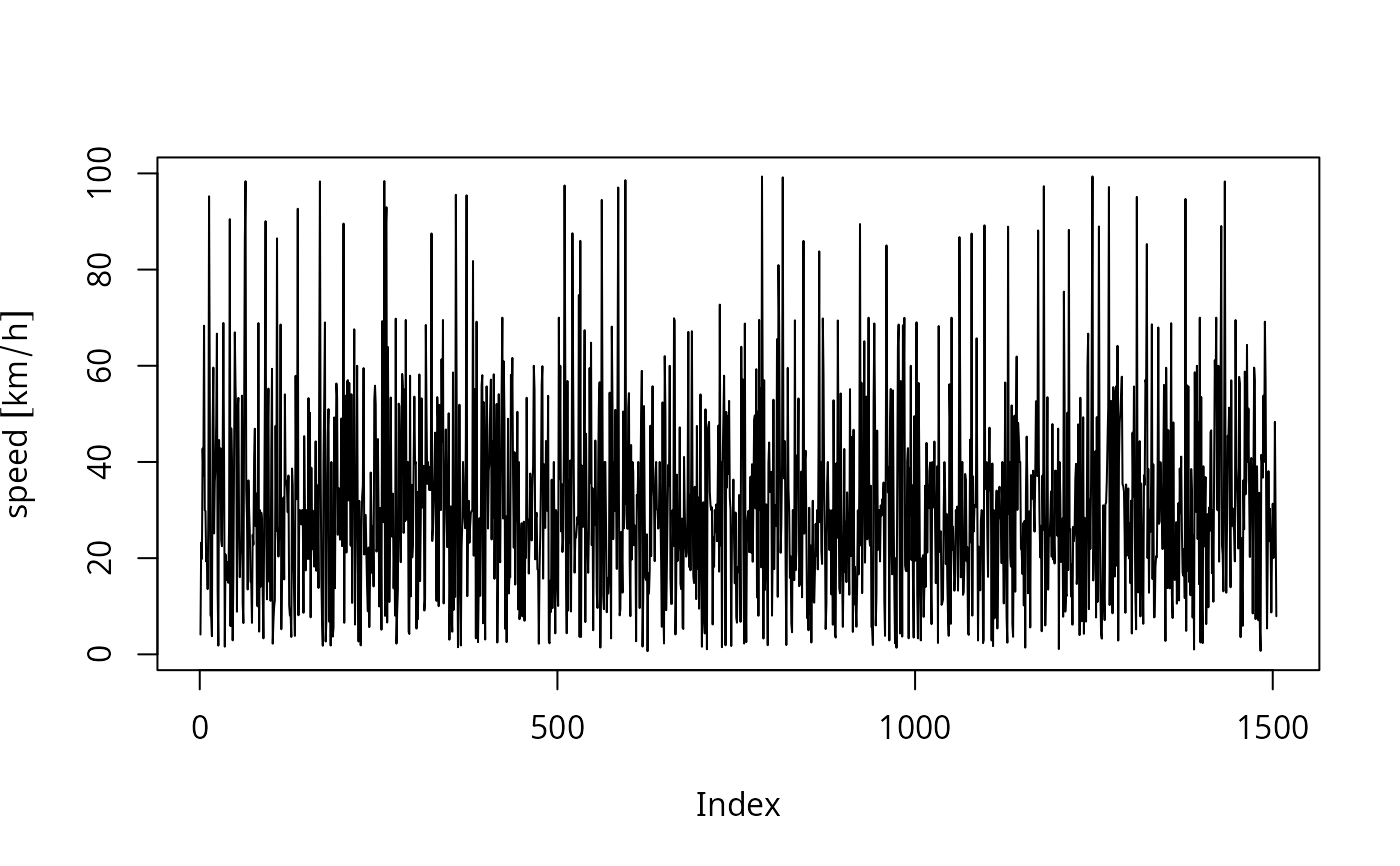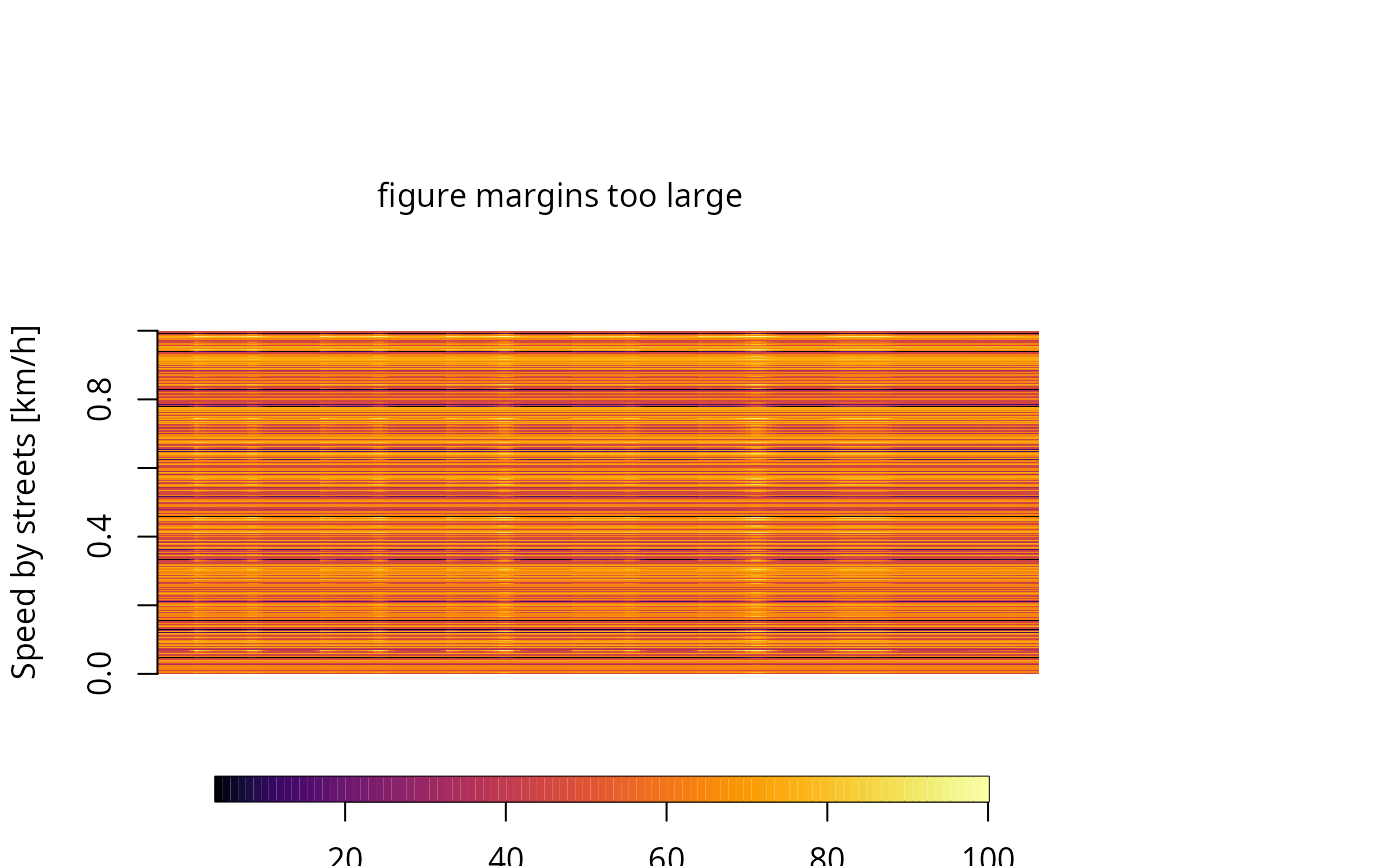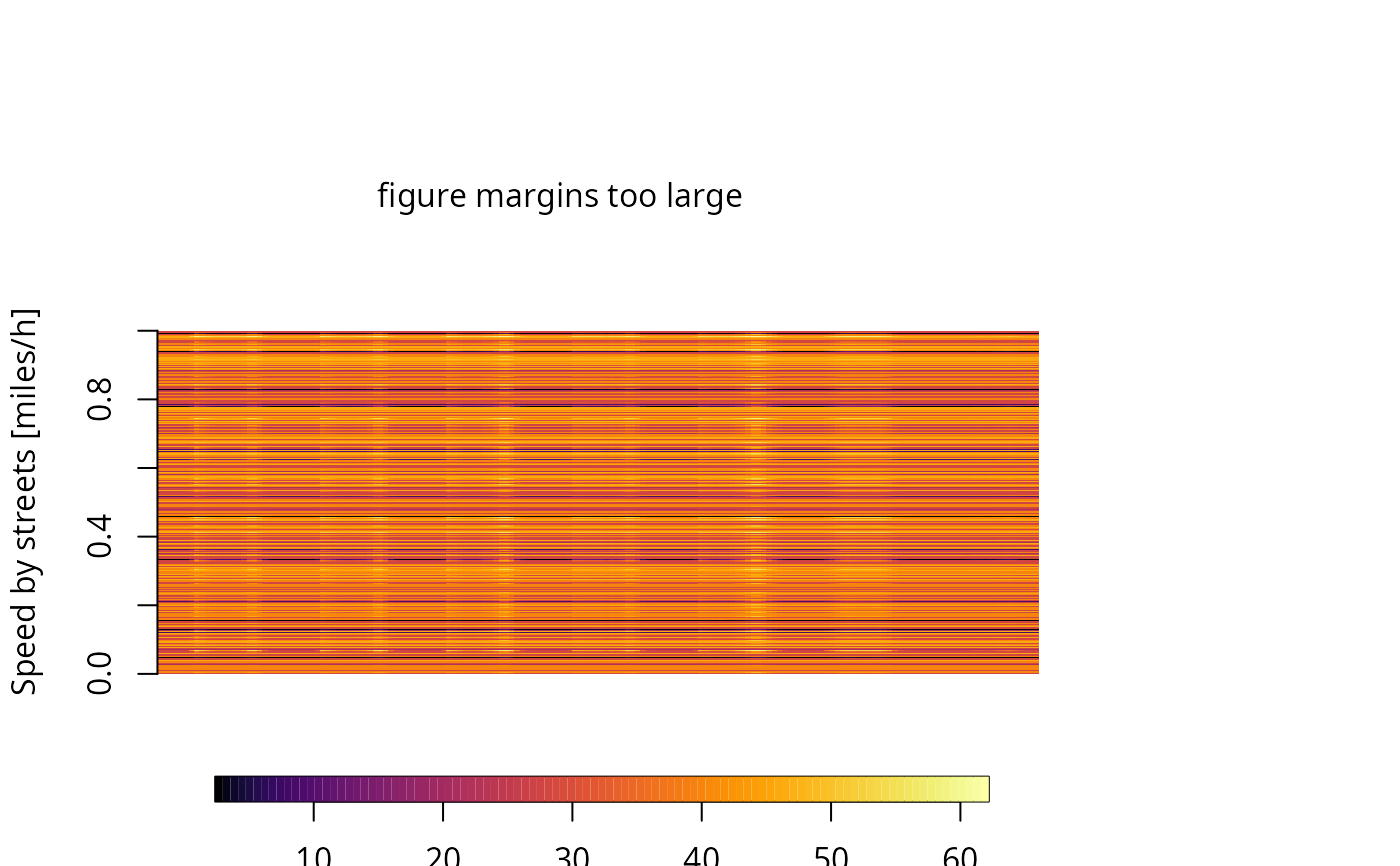Speed returns a transformed object with class "Speed" and units km/h. This function includes two arguments, distance and time. Therefore, it is possible to change the units of the speed to "m" to "s" for example. This function returns a data.frame with units for speed. When this function is applied to numeric vectors it adds class "units".

Speed(x, ..., dist = "km", time = "h")

# S3 method for Speed
print(x, ...)

# S3 method for Speed
summary(object, ...)

# S3 method for Speed
plot(
x,
pal = "mpl_inferno",
rev = FALSE,
fig1 = c(0, 0.8, 0, 0.8),
fig2 = c(0, 0.8, 0.55, 1),
fig3 = c(0.7, 1, 0, 0.8),
mai1 = c(0.2, 0.82, 0.82, 0.42),
mai2 = c(1.3, 0.82, 0.82, 0.42),
mai3 = c(0.7, 0.62, 0.82, 0.42),
bias = 1.5,
...
)

## Arguments

x

Object with class "data.frame", "matrix" or "numeric"

...

ignored Default is units is "km"

dist

String indicating the units of the resulting distance in speed.

time

Character to be the time units as denominator, default is "h"

object

Object with class "Speed"

pal

Palette of colors available or the number of the position

rev

Logical; to internally revert order of rgb color vectors.

fig1

par parameters for fig, par.

fig2

par parameters for fig, par.

fig3

par parameters for fig, par.

mai1

par parameters for mai, par.

mai2

par parameters for mai, par.

mai3

par parameters for mai, par.

bias

positive number. Higher values give more widely spaced colors at the high end.

## Value

Constructor for class "Speed" or "units"

## Note

default time unit for speed is hour

units

## Examples

{
data(net)
data(pc_profile)
speed <- Speed(net$ps) class(speed) plot(speed, type = "l") pc_week <- temp_fact(net$ldv+net$hdv, pc_profile) df <- netspeed(pc_week, net$ps, net$ffs, net$capacity, net$lkm) summary(df) plot(df) # changing to miles net$ps <- units::set_units(net$ps, "miles/h") net$ffs <- units::set_units(net$ffs, "miles/h") net$lkm <- units::set_units(net$lkm, "miles") df <- netspeed(pc_week, net$ps, net$ffs, net$capacity, net\$lkm, dist = "miles")
plot(df)
}
#> Units are the same and no cerversions will be made#> Speeds by columns and street in study area =
#>    Min. 1st Qu.  Median    Mean 3rd Qu.    Max.
#>   3.929  39.667  40.000  49.142  59.985 100.000
#> Weighted mean =  49.14#> Weighted mean =  30.54